Caribou in Covid: Contests are running online as usual. Check out the FAQ for further questions.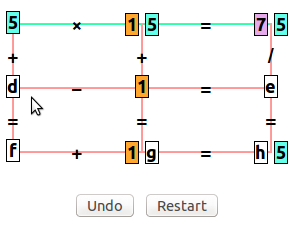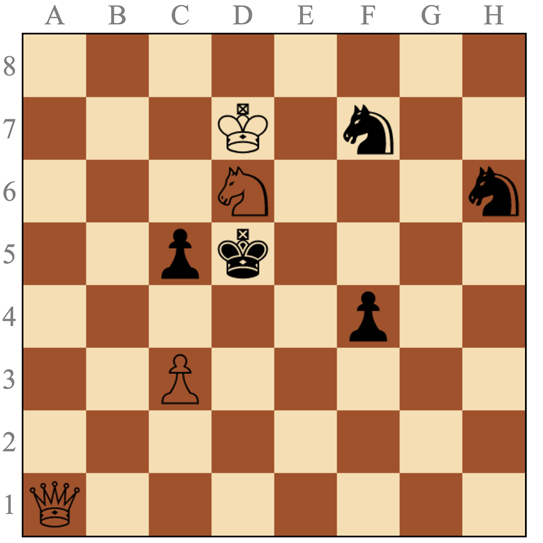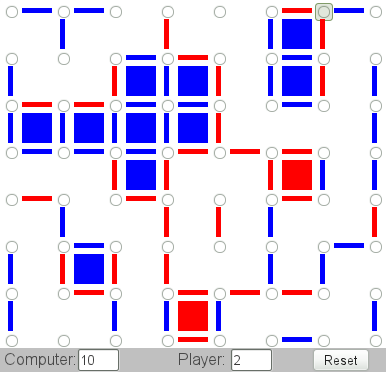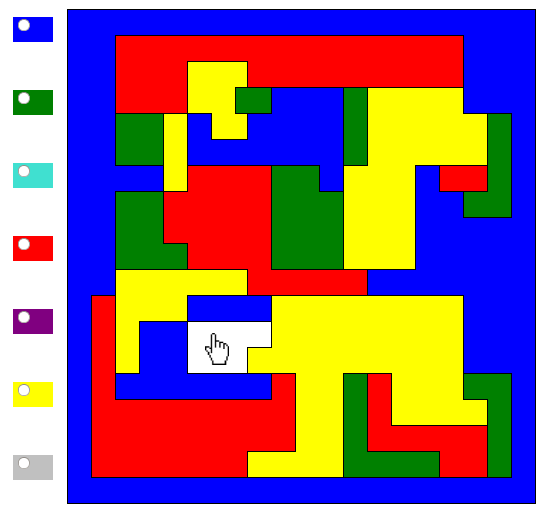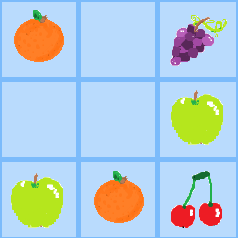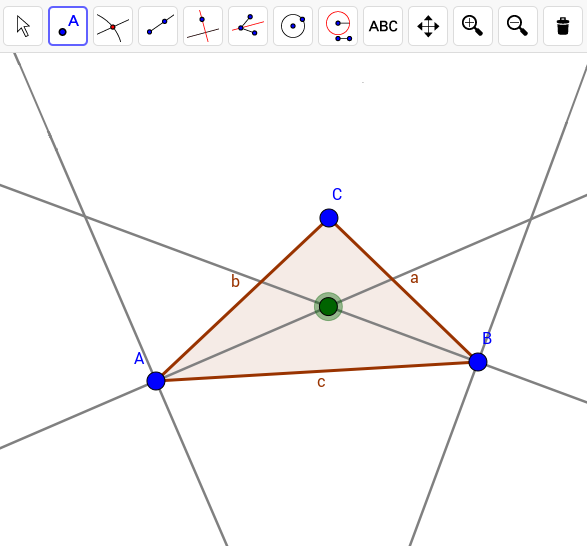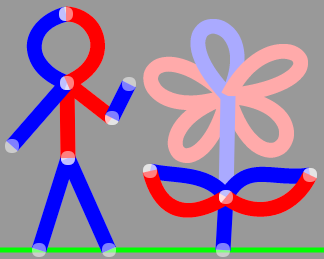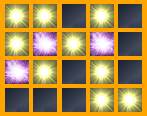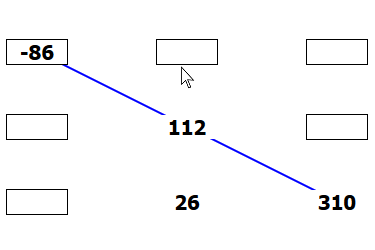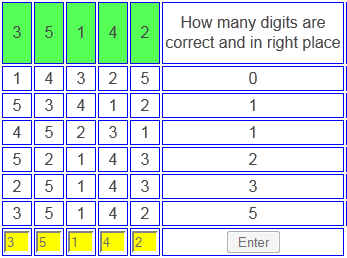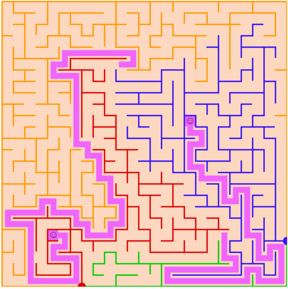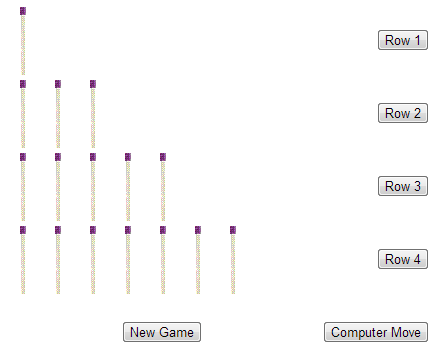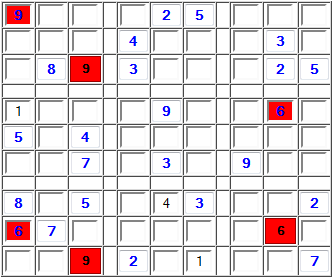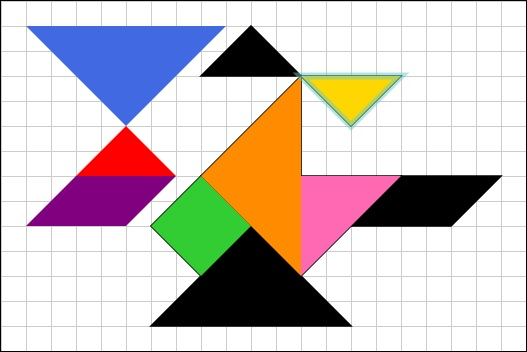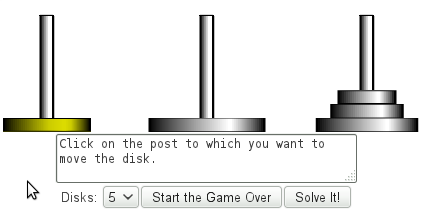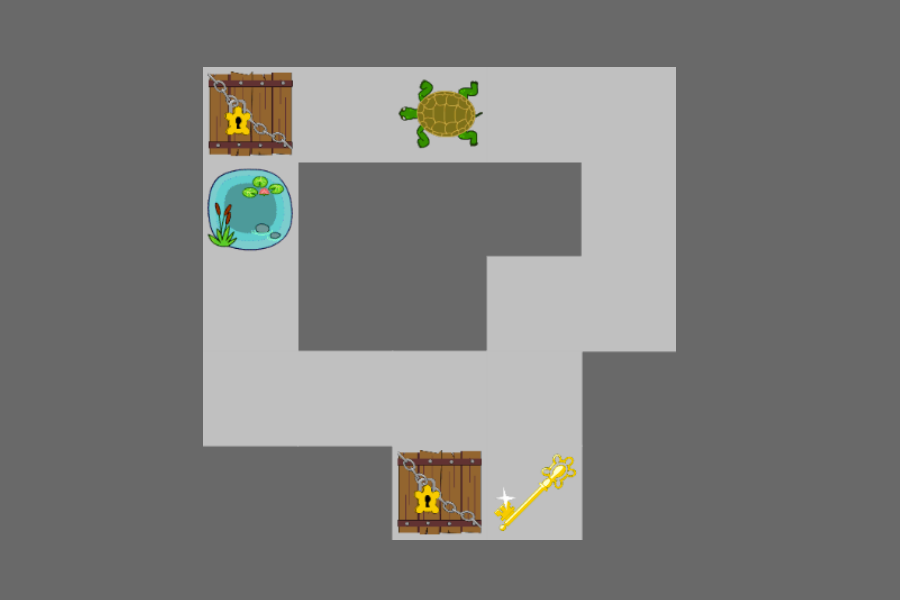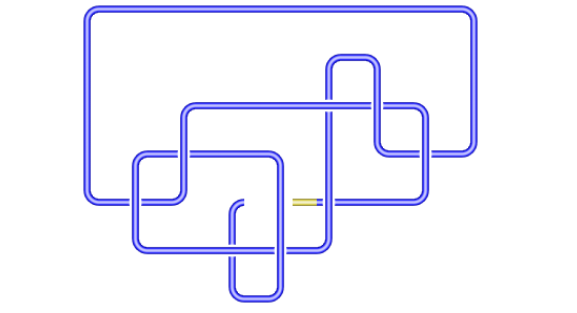## Today's Calcrostic Puzzle

```ab ×   c =  bdb
- ×   × /    +
ed ×  ec = fbcg
= =   = =    =
h × bfc = fdfb
```
What is This? | How to Solve It?

## Yesterday's Solution

```120 + 105 = 225
/ -   / /   -
8 ×  15 = 120
= =   = =   =
15 ×   7 = 105
```
Total number of plays of all games: 11,517,599